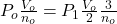Question

The volume of the vessel is reduced twice,and the number of molecules is reduced three times.How the pressure will change gas?​

1.minhkhue

P₁ = ⅔ P₀

Explanation:

For this exercise we can use the ideal gas equation

PV = n R T

let’s write the equation for the initial point, where we will use the subscript o and the end with subscript 2

Suppose the temperature does not change in the process

P₀V₀ / n₀ = P₁V₁ / n₁

They tell us that

V₁ = V₀ / 2

n₁ = n₀ / 3

we substituteP₀ =P₁

P₁ = ⅔ P₀